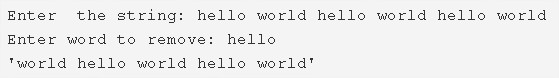# C Program To Remove First Occurrence Of A Word From String | 4 Ways

C program to remove the first occurrence of a word from a string – In this article, we will learn the several means to remove the first occurrence of a word from a string in C programming.

Suitable examples and sample programs have also been added so that you can understand the whole thing very clearly. The compiler has also been added with which you can execute it yourself.

The means used in this particular blog are as follows:

• Using Standard Method
• Using Function

A string is nothing but an array of characters. The value of a string is determined by the terminating character. Its value is considered to be 0.As you can see in the example mentioned above, you need to enter the string in the beginning.

The string entered here is as follows:

“hello world hello world hello world”

Afterwards, you need to enter the word that you want to remove.

The word “hello” is specified here for the said thing.

Hence, the final string becomes:

“world hello world hello world”

Thus, the methods to do the same in C Programming are as follows:

## Using Standard Method

1. Read the string and store into the variable ‘s’ using the function gets(s).

2) Read the entered word and save in the variable ‘w’ using gets(w).

3) For loop for(i=0;s[i];i++) iterates through the string If any element of the string is equal to white space then store the index of the white space in the integer array a[]. So a[] contains the index values of the white spaces of the string.

4) j=0, The outer for loop iterates through the index values of the white spaces of the string

a) Initialize n=a[i]-j. Here n indicates the left index of the white space.

b) If n=length of the word then

For loop iterates through the word with the structure for(l=0;w[l];l++). Compare each letter of the word with each element of the string as s[i+l]==w[l]. If both the elements are equal then increase t value. Repeat this step till the last element of the word. If t is equal to the length of the word then break the outer for loop.

If the word has not occurred then initialize j=a[i]+l and go to the next white space. Repeat until finds the first occurrence of the word.

5) To remove the first occurrence of the word

j=a[i]+1 j indicates the index of the first occurrence of the word.

l=0,i=j+t+1.Here i indicates the index of the next word to the removed word.

a) Shift the elements right to the first occurrence of the word, to left as follow

While loop iterates until the last element of the string become to null.

s[j+l]=s[j+t+l+1] by increasing l value.

6) After removing the first occurrence of the word, print the string. Now the length of the string=length of the original string-length of the word.

Output:

### Using Function

1. The main() calls the check(char *s, char *w) to remove the first occurrence of the word from the given string.

a) Find the index values of the white spaces and save the values in the integer array a[]. Initialize the last iteration value of i as last index value in the array a[].

b) j=0, iterate through the white spaces,

Initialize n=a[i]-j

length of the word is equal to n then compare the elements of the word with elements of the string. If matched then t++.Repeat for all elements of the word. If t=word length then breaks the “for loop”.

If t not equal to word length then j=index of the next white space+1, and go through the next whitespace(step b). Repeat until finds the first occurrence of the word.

c) Shift the elements right to the first occurrence of the word, to  “length of the word+index of the whitespace next to the first occurrence of the word” positions left.

Output:

x

## C Program To Check If Alphabet, Digit or Special Character | C Programs

C program to check for an alphabet, digit or special character – In this article, ...